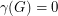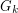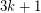Importance: High ✭✭✭
 Author(s): Chudnovsky, Maria Seymour, Paul D. Sullivan, Blair
 Subject: Graph Theory » Directed Graphs
 Keywords: acyclic digraph feedback edge set triangle free
 Posted by: mdevos on: July 8th, 2008

For any simple digraph, we letbe the number of unordered pairs of nonadjacent vertices (i.e. the number of non-edges), andbe the size of the smallest feedback edge set.

Conjecture  Ifis a simple digraph without directed cycles of length, then.

Ifsatisfies, thenis a tournament, and it is easy to check thatwill have a directed cycle of length three unless it is acyclic, in which case. So in this case, the conjecture holds. More generally, it is natural to suspect that a digraph with few non-edges and no directed triangles should be close to acyclic. Indeed, this conjecture asserts a precise relationship of this form.

If true, the above conjecture is essentially tight for a number of examples. We noted above that it is tight for transitive tournaments. Here is another basic class: letbe the circulant digraph obtained by placingvertices in a circle, and adding an edge directed fromtowheneveris distancefromin the clockwise order. Such examples may be nested to obtain new ones.

Chudnovsky, Seymour, and Sullivan [CSS] utilized a clever double counting argument to prove thatalways holds. They also proved their conjecture in the case whenis the union of two cliques, and whenis a circular interval digraph.

## Bibliography

*[CSS] M. Chudnovsky, P.D. Seymour, and B. Sullivan, Cycles in dense digraphs.

* indicates original appearance(s) of problem.